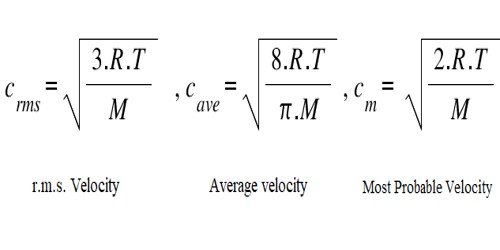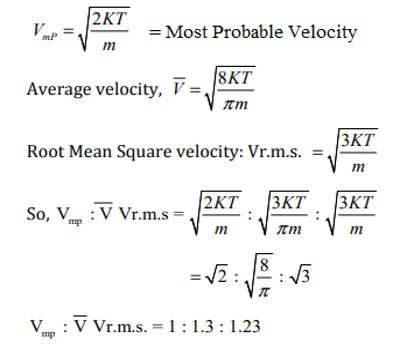# Average Velocity, r.m.s. Velocity and Most Probable Velocity: Equations

Average velocity is defined as disarticulation (i.e., change in position) per unit time. This velocity is disarticulation divided by a time period of the disarticulation. Root mean square (RMS) velocity is the (square root of the) average value of the square of its velocity. The first typical velocity is easiest to estimate and term as the most probable velocity.

Average Velocity, r.m.s. Velocity and Most Probable Velocity relationship –

The distribution equation [dn/n = 4π (M/2πRT)3/2 x e– (mc2/2RT) x c2dc]; may be used 10 obtain the average velocity, c , of the molecules.

This is given by the relation:

c = √(8RT/πM)

If we compare the average velocity with r.m.s. velocity may be seen that values are not the same,

Average velocity: C = √(8/3π) x r.m.s. velocity = 0.9213 x r.m.s. velocity

Most probable velocity is the velocity possessed by the largest number of molecules in a gas. Maxwell showed that the most probable velocity is given by the expression.

Cmpv = √(2RT/M)

A value of the most probable velocity may be calculated from the values of R, T, and M. A relation between the r.m s. velocity and the most probable velocity can be established as follows:

Cmpv/Cr.m.s. = [√(2RT/M)/√3RT/M] = √2/3 = 0.8165

Hence, Cmpv = 0.8165 x Cr.m.s

Average velocity

The average velocity of an object is its total disarticulation divided by the entire time taken. In other words, it is the rate at which an object changes its position from one place to another. It is a Vector quantity. The SI unit is meters per second. However, any distance unit per any time unit can be used when necessary, such as miles per hour (mph) or kilometer per hour (kmph).

The average velocity formula: average velocity = (change in position) / (change in time)

If we were given a position function, s(t), which gave the position of an object at time t, then the average velocity between time t sub 1 and t sub 2 is given by the formula:

Vavg = [s(t2) – s(t1)] / (t2 – t1) or, Δd/Δt.

Root mean square velocity

RMS velocity is the square root of the mean of squares of the speed of individual gas molecules. The root-mean-square velocity is that of a wave through subsurface layers of dissimilar interval velocity along a particular ray path and is usually quite a few percents higher than the average velocity. The stacking velocity and the root-mean-square velocity approach equality when source-receiver offset approaches zero and layers are horizontal and isotropic.

Root mean square speed = √(3RT/M)

The most probable velocity

The most probable velocity at which the most molecules in gas travel. The first distinctive velocity is easiest to estimate and term as the most probable velocity. The formula for most probable velocity is, Mp = √(2RT/M).Fig: Average Velocity, r.m.s. Velocity and Most Probable Velocity – a relationship in equation